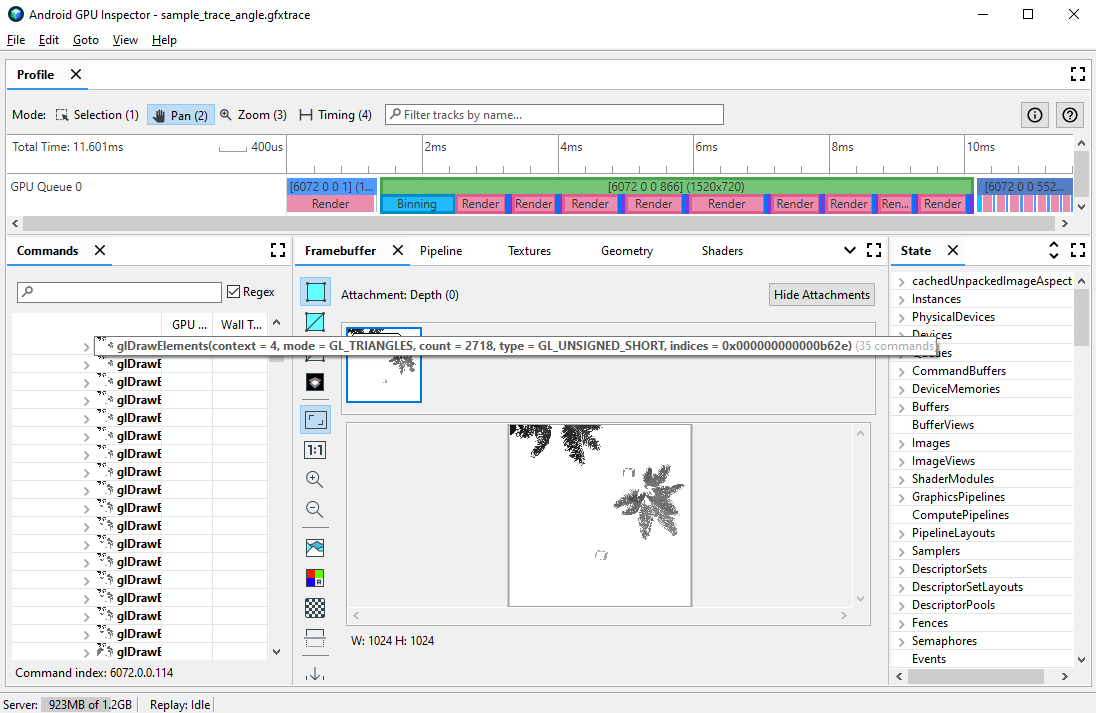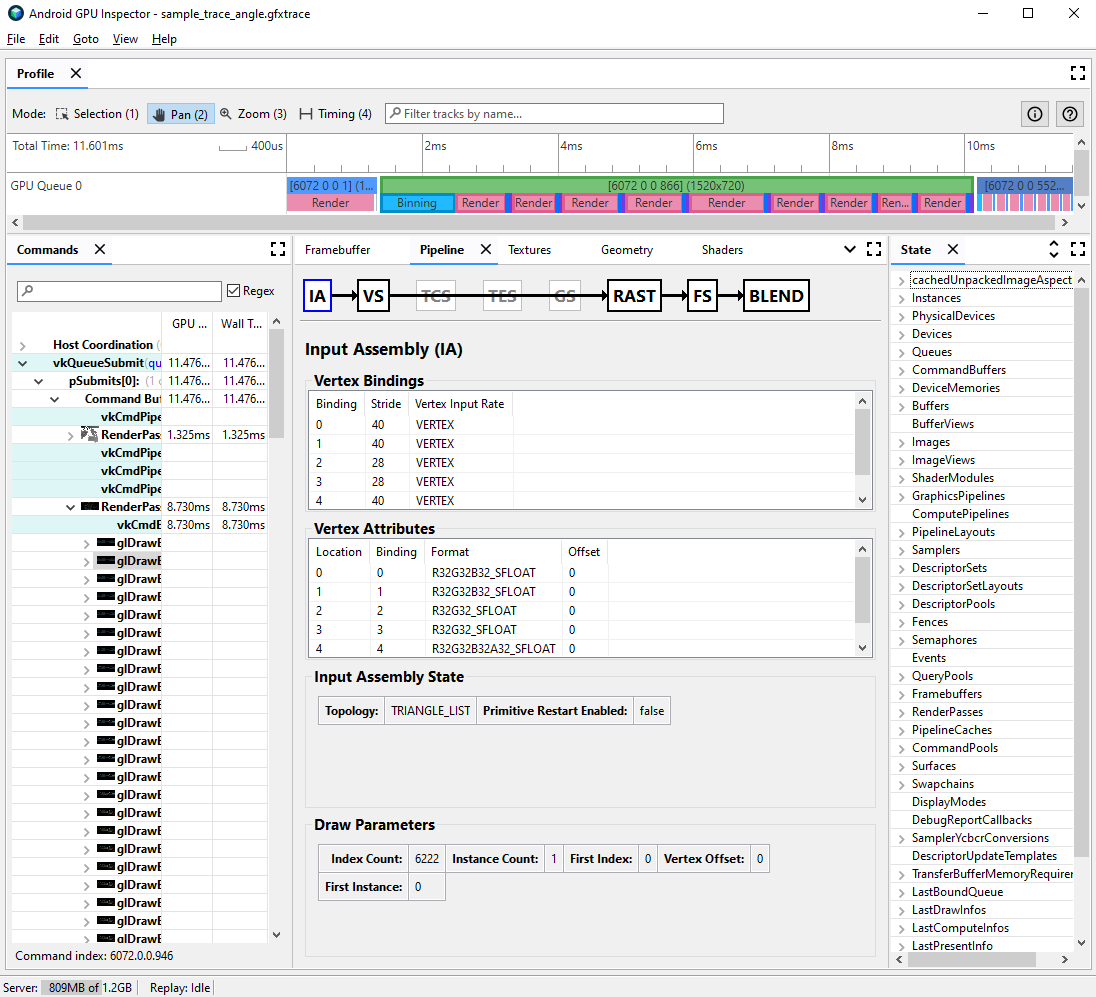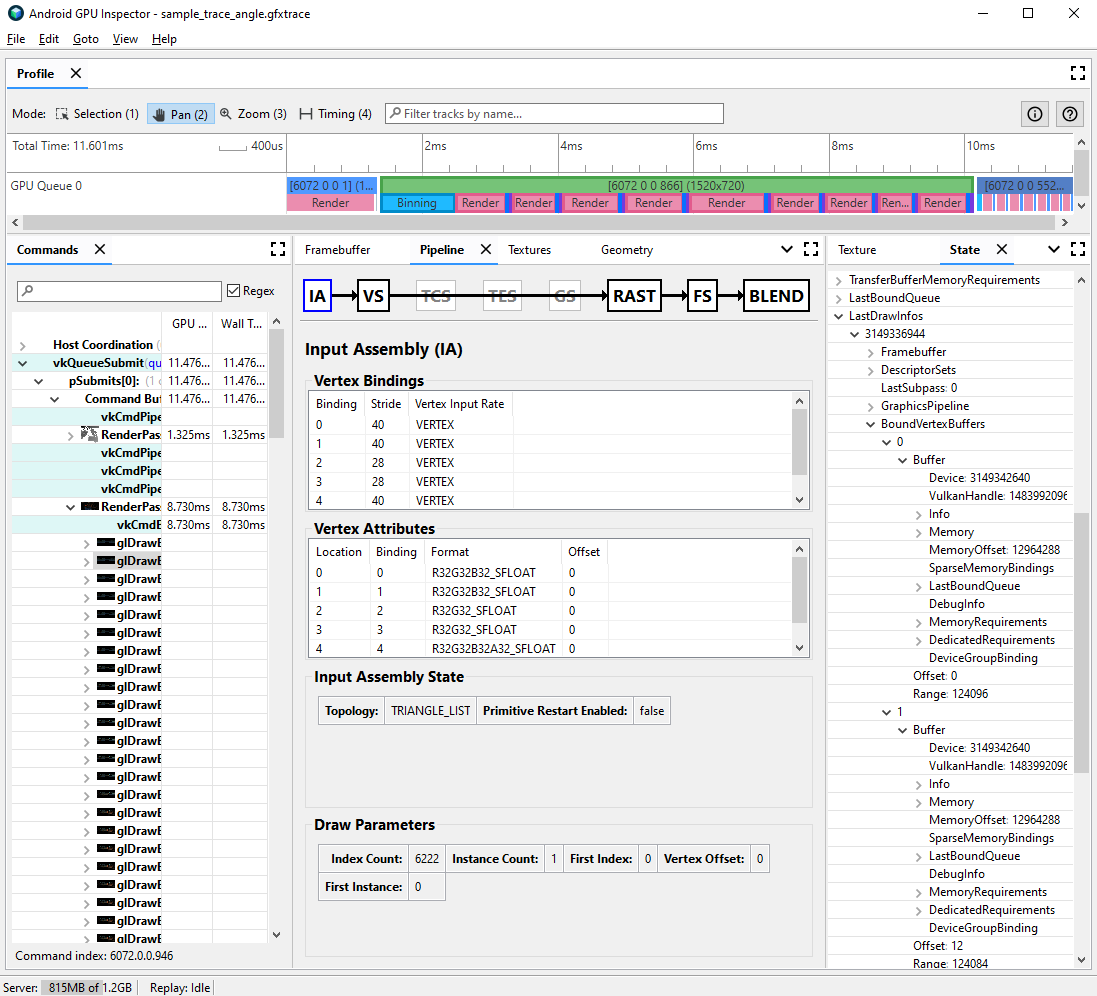# Analyze vertex formats

You may diagnose a few possible vertex-related performance problems through the use of frame profiling. Use the Commands pane to view all of the draw calls your game performs in a given frame and counts of primitives drawn per draw call. This can give an approximation of the overall number of vertices submitted in a single frame.Figure 1. Frame profiling view for a single glDrawElements call, showing 2,718 triangle primitives drawn

## Vertex attribute compression

One common problem your game may face is a large average vertex size. A large number of vertices submitted with a high average vertex size results in a large vertex memory read bandwidth when read by the GPU.

To observe the vertex format for a given draw call, complete the following steps:

1. Select a draw call of interest.

This can be a typical draw call for the scene, a draw call with a large number of vertices, a draw call for a complex character model, or some other type of draw call.

2. Navigate to the Pipeline pane, and click IA for input assembly. This defines the vertex format for vertices coming into the GPU.

3. Observe a series of attributes and their formats; for example, `R32G32B32_SFLOAT` is a 3-component 32-bit signed float.Figure 2. Input assembly for a draw call, with uncompressed attributes resulting in a vertex size of 56 bytes

Frequently, vertex attributes can be compressed with minimal reduction in the quality of the models drawn. In particular, we recommend:

• Compressing vertex position to half-precision 16-bit floats
• Compressing UV texture coordinates to 16-bit unsigned integer ushorts
• Compressing the tangent space by encoding normal, tangent, and binormal vectors using quaternions

Other miscellaneous attributes may also be considered for lower-precision types on a case-by-case basis.

## Vertex stream splitting

You can also investigate whether vertex attribute streams are appropriately split. On tiled rendering architectures such as mobile GPUs, vertex positions are first used in a binning pass to create bins of primitives processed in each tile. If vertex attributes are interleaved into a single buffer, all vertex data is read into cache for binning, even though only vertex positions are used.

To reduce vertex read memory bandwidth and improve cache efficiency, and thus reduce time spent on the binning pass, vertex data should be split into two separate streams, one for vertex positions, and one for all other vertex attributes.

To investigate whether vertex attributes are appropriately split:

1. Select a draw call of interest, and note the draw call number.

This can be a typical draw call for the scene, a draw call with a large number of vertices, a draw call for a complex character model, or some other type of draw call.

2. Navigate to the Pipeline pane, and click IA for input assembly. This defines the vertex format for vertices coming into the GPU.

3. Observe the bindings of your vertex attributes; typically these might increase linearly (0, 1, 2, 3, etc.), but this is not always the case. Vertex position is typically the first vertex attribute listed.

4. In the State pane, find the `LastDrawInfos` and expand the matching draw call number. Then, expand the `BoundVertexBuffers` for this draw call.

5. Observe the vertex buffers bound during the given draw call, with indices matching the vertex attribute bindings from earlier.

6. Expand the bindings for your draw call’s vertex attributes, and expand the buffers.

7. Observe the `VulkanHandle` for the buffers, which represent the underlying memory that the vertex data sources from. If the `VulkanHandle`s are different, this means the attributes originate from different underlying buffers. If the `VulkanHandle`s are the same but the offsets are large (for example, greater than 100), the attributes may still originate from different sub-buffers, but this requires further investigation.Figure 3. Input assembly for a draw call, with the state panel to the right showing that the attributes at binding 0 and 1, vertex position and normal, share a single underlying buffer

For more detail about vertex stream splitting and how to resolve it on various game engines, see our blog post on the subject.

[]
[]